Dr. S. R. Lasker Library Online Catalogue

Normal view

# Mathematical statistics with applications / Dennis D. Wackerly, William Mendenhall III, Richard L. Scheaffer.

Material type:TextLanguage: English Publication details: c2008. Edition: 7th ed. ; international student edDescription: xxii, 912 p. : ill., tables ; 24 cmISBN: 9780495385080; 0495385085Subject(s): Mathematical statisticsDDC classification: 519.5 MAT Online resources: OCLC | Ebook Fulltext
Contents:
1. What Is Statistics? Introduction. Characterizing a Set of Measurements: Graphical Methods. Characterizing a Set of Measurements: Numerical Methods. How Inferences Are Made. Theory and Reality. Summary. 2. Probability. Introduction. Probability and Inference. A Review of Set Notation. A Probabilistic Model for an Experiment: The Discrete Case. Calculating the Probability of an Event: The Sample-Point Method. Tools for Counting Sample Points. Conditional Probability and the Independence of Events. Two Laws of Probability. Calculating the Probability of an Event: The Event-Composition Methods. The Law of Total Probability and Bayes"s Rule. Numerical Events and Random Variables. Random Sampling. Summary. 3. Discrete Random Variables and Their Probability Distributions. Basic Definition. The Probability Distribution for Discrete Random Variable. The Expected Value of Random Variable or a Function of Random Variable. The Binomial Probability Distribution. The Geometric Probability Distribution. The Negative Binomial Probability Distribution (Optional). The Hypergeometric Probability Distribution. Moments and Moment-Generating Functions. Probability-Generating Functions (Optional). Tchebysheff"s Theorem. Summary. 4. Continuous Random Variables and Their Probability Distributions. Introduction. The Probability Distribution for Continuous Random Variable. The Expected Value for Continuous Random Variable. The Uniform Probability Distribution. The Normal Probability Distribution. The Gamma Probability Distribution. The Beta Probability Distribution. Some General Comments. Other Expected Values. Tchebysheff"s Theorem. Expectations of Discontinuous Functions and Mixed Probability Distributions (Optional). Summary. 5. Multivariate Probability Distributions. Introduction. Bivariate and Multivariate Probability Distributions. Independent Random Variables. The Expected Value of a Function of Random Variables. Special Theorems. The Covariance of Two Random Variables. The Expected Value and Variance of Linear Functions of Random Variables. The Multinomial Probability Distribution. The Bivariate Normal Distribution (Optional). Conditional Expectations. Summary. 6. Functions of Random Variables. Introductions. Finding the Probability Distribution of a Function of Random Variables. The Method of Distribution Functions. The Methods of Transformations. Multivariable Transformations Using Jacobians. Order Statistics. Summary. 7. Sampling Distributions and the Central Limit Theorem. Introduction. Sampling Distributions Related to the Normal Distribution. The Central Limit Theorem. A Proof of the Central Limit Theorem (Optional). The Normal Approximation to the Binomial Distributions. Summary. 8. Estimation. Introduction. The Bias and Mean Square Error of Point Estimators. Some Common Unbiased Point Estimators. Evaluating the Goodness of Point Estimator. Confidence Intervals. Large-Sample Confidence Intervals Selecting the Sample Size. Small-Sample Confidence Intervals for u and u1-u2. Confidence Intervals for o2. Summary. 9. Properties of Point Estimators and Methods of Estimation. Introduction. Relative Efficiency. Consistency. Sufficiency. The Rao-Blackwell Theorem and Minimum-Variance Unbiased Estimation. The Method of Moments. The Method of Maximum Likelihood. Some Large-Sample Properties of MLEs (Optional). Summary. 10. Hypothesis Testing. Introduction. Elements of a Statistical Test. Common Large-Sample Tests. Calculating Type II Error Probabilities and Finding the Sample Size for the Z Test. Relationships Between Hypothesis Testing Procedures and Confidence Intervals. Another Way to Report the Results of a Statistical Test: Attained Significance Levels or p-Values. Some Comments on the Theory of Hypothesis Testing. Small-Sample Hypothesis Testing for u and u1-u2. Testing Hypotheses Concerning Variances. Power of Test and the Neyman-Pearson Lemma. Likelihood Ration Test. Summary. 11. Linear Models and Estimation by Least Squares. Introduction. Linear Statistical Models. The Method of Least Squares. Properties of the Least Squares Estimators for the Simple Linear Regression Model. Inference Concerning the Parameters BI. Inferences Concerning Linear Functions of the Model Parameters: Simple Linear Regression. Predicting a Particular Value of Y Using Simple Linear Regression. Correlation. Some Practical Examples. Fitting the Linear Model by Using Matrices. Properties of the Least Squares Estimators for the Multiple Linear Regression Model. Inferences Concerning Linear Functions of the Model Parameters: Multiple Linear Regression. Prediction a Particular Value of Y Using Multiple Regression. A Test for H0: Bg+1 + Bg+2 = . = Bk = 0. Summary and Concluding Remarks. 12. Considerations in Designing Experiments. The Elements Affecting the Information in a Sample. Designing Experiment to Increase Accuracy. The Matched Pairs Experiment. Some Elementary Experimental Designs. Summary. 13. The Analysis of Variance. Introduction. The Analysis of Variance Procedure. Comparison of More than Two Means: Analysis of Variance for a One-way Layout. An Analysis of Variance Table for a One-Way Layout. A Statistical Model of the One-Way Layout. Proof of Additivity of the Sums of Squares and E (MST) for a One-Way Layout (Optional). Estimation in the One-Way Layout. A Statistical Model for the Randomized Block Design. The Analysis of Variance for a Randomized Block Design. Estimation in the Randomized Block Design. Selecting the Sample Size. Simultaneous Confidence Intervals for More than One Parameter. Analysis of Variance Using Linear Models. Summary. 14. Analysis of Categorical Data. A Description of the Experiment. The Chi-Square Test. A Test of Hypothesis Concerning Specified Cell Probabilities: A Goodness-of-Fit Test. Contingency Tables. r x c Tables with Fixed Row or Column Totals. Other Applications. Summary and Concluding Remarks. 15. Nonparametric Statistics. Introduction. A General Two-Sampling Shift Model. A Sign Test for a Matched Pairs Experiment. The Wilcoxon Signed-Rank Test for a Matched Pairs Experiment. The Use of Ranks for Comparing Two Population Distributions: Independent Random Samples. The Mann-Whitney U Test: Independent Random Samples. The Kruskal-Wallis Test for One-Way Layout. The Friedman Test for Randomized Block Designs. The Runs Test: A Test for Randomness. Rank Correlation Coefficient. Some General Comments on Nonparametric Statistical Test. 16. Introduction to Bayesian Methods for Inference. Introduction. Bayesian Priors, Posteriors and Estimators. Bayesian Credible Intervals. Bayesian Tests of Hypotheses. Summary and Additional Comments. Appendix 1. Matrices and Other Useful Mathematical Results. Matrices and Matrix Algebra. Addition of Matrices. Multiplication of a Matrix by a Real Number. Matrix Multiplication. Identity Elements. The Inverse of a Matrix. The Transpose of a Matrix. A Matrix Expression for a System of Simultaneous Linear Equations. Inverting a Matrix. Solving a System of Simultaneous Linear Equations. Other Useful Mathematical Results. Appendix 2. Common Probability Distributions, Means, Variances, and Moment-Generating Functions. Discrete Distributions. Continuous Distributions. Appendix 3. Tables. Binomial Probabilities. Table of e-x. Poisson Probabilities. Normal Curve Areas. Percentage Points of the t Distributions. Percentage Points of the F Distributions. Distribution of Function U. Critical Values of T in the Wilcoxon Matched-Pairs, Signed-Ranks Test. Distribution of the Total Number of Runs R in Sample Size (n1,n2); P(R < a). Critical Values of Pearman's Rank Correlation Coefficient. Random Numbers. Answer to Exercises. Index.
Summary: In their bestselling MATHEMATICAL STATISTICS WITH APPLICATIONS, premiere authors Dennis Wackerly, William Mendenhall, and Richard L. Scheaffer present a solid foundation in statistical theory while conveying the relevance and importance of the theory in solving practical problems in the real world. The authors' use of practical applications and excellent exercises helps you discover the nature of statistics and understand its essential role in scientific research.
Tags from this library: No tags from this library for this title.
Star ratingsAverage rating: 0.0 (0 votes)
Holdings
Item type Current library Collection Call number Copy number Status Date due Barcode Item holdsE-Book
E-book
Non-fiction 519.5 MAT 2008 (Browse shelf(Opens below)) Not for loanText
Reserve Section
Non-fiction 519.5 MAT 2008 (Browse shelf(Opens below)) C-1 Not For Loan 25368Text
Circulation Section
Non-fiction 519.5 MAT 2008 (Browse shelf(Opens below)) C-2 Available 25369Text
Circulation Section
Non-fiction 519.5 MAT 2008 (Browse shelf(Opens below)) C-3 Available 25370
Total holds: 0
##### Browsing EWU Library shelves, Shelving location: E-book Close shelf browser (Hides shelf browser)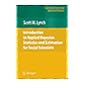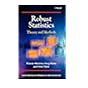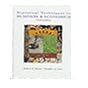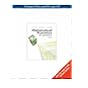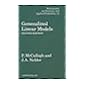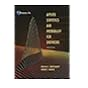519.5 LYI 2007 Introduction to applied Bayesian statistics and estimation for social scientists / 519.5 MAR 2006 Robust statistics : 519.5 MAS 1996 Statistical techniques in business and economics / 519.5 MAT 2008 Mathematical statistics with applications / 519.5 MCG 1989 Generalized linear models / 519.5 MIJ 2014 John E. Freund's mathematical statistics with applications / 519.5 MOA 2003 Applied statistics and probability for engineers /

Includes bibliographical references and index.

1. What Is Statistics? Introduction. Characterizing a Set of Measurements: Graphical Methods. Characterizing a Set of Measurements: Numerical Methods. How Inferences Are Made. Theory and Reality. Summary. 2. Probability. Introduction. Probability and Inference. A Review of Set Notation. A Probabilistic Model for an Experiment: The Discrete Case. Calculating the Probability of an Event: The Sample-Point Method. Tools for Counting Sample Points. Conditional Probability and the Independence of Events. Two Laws of Probability. Calculating the Probability of an Event: The Event-Composition Methods. The Law of Total Probability and Bayes"s Rule. Numerical Events and Random Variables. Random Sampling. Summary. 3. Discrete Random Variables and Their Probability Distributions. Basic Definition. The Probability Distribution for Discrete Random Variable. The Expected Value of Random Variable or a Function of Random Variable. The Binomial Probability Distribution. The Geometric Probability Distribution. The Negative Binomial Probability Distribution (Optional). The Hypergeometric Probability Distribution. Moments and Moment-Generating Functions. Probability-Generating Functions (Optional). Tchebysheff"s Theorem. Summary. 4. Continuous Random Variables and Their Probability Distributions. Introduction. The Probability Distribution for Continuous Random Variable. The Expected Value for Continuous Random Variable. The Uniform Probability Distribution. The Normal Probability Distribution. The Gamma Probability Distribution. The Beta Probability Distribution. Some General Comments. Other Expected Values. Tchebysheff"s Theorem. Expectations of Discontinuous Functions and Mixed Probability Distributions (Optional). Summary. 5. Multivariate Probability Distributions. Introduction. Bivariate and Multivariate Probability Distributions. Independent Random Variables. The Expected Value of a Function of Random Variables. Special Theorems. The Covariance of Two Random Variables. The Expected Value and Variance of Linear Functions of Random Variables. The Multinomial Probability Distribution. The Bivariate Normal Distribution (Optional). Conditional Expectations. Summary. 6. Functions of Random Variables. Introductions. Finding the Probability Distribution of a Function of Random Variables. The Method of Distribution Functions. The Methods of Transformations. Multivariable Transformations Using Jacobians. Order Statistics. Summary. 7. Sampling Distributions and the Central Limit Theorem. Introduction. Sampling Distributions Related to the Normal Distribution. The Central Limit Theorem. A Proof of the Central Limit Theorem (Optional). The Normal Approximation to the Binomial Distributions. Summary. 8. Estimation. Introduction. The Bias and Mean Square Error of Point Estimators. Some Common Unbiased Point Estimators. Evaluating the Goodness of Point Estimator. Confidence Intervals. Large-Sample Confidence Intervals Selecting the Sample Size. Small-Sample Confidence Intervals for u and u1-u2. Confidence Intervals for o2. Summary. 9. Properties of Point Estimators and Methods of Estimation. Introduction. Relative Efficiency. Consistency. Sufficiency. The Rao-Blackwell Theorem and Minimum-Variance Unbiased Estimation. The Method of Moments. The Method of Maximum Likelihood. Some Large-Sample Properties of MLEs (Optional). Summary. 10. Hypothesis Testing. Introduction. Elements of a Statistical Test. Common Large-Sample Tests. Calculating Type II Error Probabilities and Finding the Sample Size for the Z Test. Relationships Between Hypothesis Testing Procedures and Confidence Intervals. Another Way to Report the Results of a Statistical Test: Attained Significance Levels or p-Values. Some Comments on the Theory of Hypothesis Testing. Small-Sample Hypothesis Testing for u and u1-u2. Testing Hypotheses Concerning Variances. Power of Test and the Neyman-Pearson Lemma. Likelihood Ration Test. Summary. 11. Linear Models and Estimation by Least Squares. Introduction. Linear Statistical Models. The Method of Least Squares. Properties of the Least Squares Estimators for the Simple Linear Regression Model. Inference Concerning the Parameters BI. Inferences Concerning Linear Functions of the Model Parameters: Simple Linear Regression. Predicting a Particular Value of Y Using Simple Linear Regression. Correlation. Some Practical Examples. Fitting the Linear Model by Using Matrices. Properties of the Least Squares Estimators for the Multiple Linear Regression Model. Inferences Concerning Linear Functions of the Model Parameters: Multiple Linear Regression. Prediction a Particular Value of Y Using Multiple Regression. A Test for H0: Bg+1 + Bg+2 = . = Bk = 0. Summary and Concluding Remarks. 12. Considerations in Designing Experiments. The Elements Affecting the Information in a Sample. Designing Experiment to Increase Accuracy. The Matched Pairs Experiment. Some Elementary Experimental Designs. Summary. 13. The Analysis of Variance. Introduction. The Analysis of Variance Procedure. Comparison of More than Two Means: Analysis of Variance for a One-way Layout. An Analysis of Variance Table for a One-Way Layout. A Statistical Model of the One-Way Layout. Proof of Additivity of the Sums of Squares and E (MST) for a One-Way Layout (Optional). Estimation in the One-Way Layout. A Statistical Model for the Randomized Block Design. The Analysis of Variance for a Randomized Block Design. Estimation in the Randomized Block Design. Selecting the Sample Size. Simultaneous Confidence Intervals for More than One Parameter. Analysis of Variance Using Linear Models. Summary. 14. Analysis of Categorical Data. A Description of the Experiment. The Chi-Square Test. A Test of Hypothesis Concerning Specified Cell Probabilities: A Goodness-of-Fit Test. Contingency Tables. r x c Tables with Fixed Row or Column Totals. Other Applications. Summary and Concluding Remarks. 15. Nonparametric Statistics. Introduction. A General Two-Sampling Shift Model. A Sign Test for a Matched Pairs Experiment. The Wilcoxon Signed-Rank Test for a Matched Pairs Experiment. The Use of Ranks for Comparing Two Population Distributions: Independent Random Samples. The Mann-Whitney U Test: Independent Random Samples. The Kruskal-Wallis Test for One-Way Layout. The Friedman Test for Randomized Block Designs. The Runs Test: A Test for Randomness. Rank Correlation Coefficient. Some General Comments on Nonparametric Statistical Test. 16. Introduction to Bayesian Methods for Inference. Introduction. Bayesian Priors, Posteriors and Estimators. Bayesian Credible Intervals. Bayesian Tests of Hypotheses. Summary and Additional Comments. Appendix 1. Matrices and Other Useful Mathematical Results. Matrices and Matrix Algebra. Addition of Matrices. Multiplication of a Matrix by a Real Number. Matrix Multiplication. Identity Elements. The Inverse of a Matrix. The Transpose of a Matrix. A Matrix Expression for a System of Simultaneous Linear Equations. Inverting a Matrix. Solving a System of Simultaneous Linear Equations. Other Useful Mathematical Results. Appendix 2. Common Probability Distributions, Means, Variances, and Moment-Generating Functions. Discrete Distributions. Continuous Distributions. Appendix 3. Tables. Binomial Probabilities. Table of e-x. Poisson Probabilities. Normal Curve Areas. Percentage Points of the t Distributions. Percentage Points of the F Distributions. Distribution of Function U. Critical Values of T in the Wilcoxon Matched-Pairs, Signed-Ranks Test. Distribution of the Total Number of Runs R in Sample Size (n1,n2); P(R < a). Critical Values of Pearman's Rank Correlation Coefficient. Random Numbers. Answer to Exercises. Index.

In their bestselling MATHEMATICAL STATISTICS WITH APPLICATIONS, premiere authors Dennis Wackerly, William Mendenhall, and Richard L. Scheaffer present a solid foundation in statistical theory while conveying the relevance and importance of the theory in solving practical problems in the real world. The authors' use of practical applications and excellent exercises helps you discover the nature of statistics and understand its essential role in scientific research.

AS

Tahur Ahmed

There are no comments on this title.